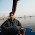Here you can find a number of examples on Java Script.Please find the various kinds of examples listed on the right side of this site. If you don't find anything on Java Script then please write to us or leave a commnet. We are pleased to help and find the solution of your problem.

### JavaScript: Squareroot Function

sqrt Function :-
Returns the square root of a number.

Suntax :-
sqrt(number)

Description :-
If the value of `number` is negative,`sqrt` returns `NaN`. Because `sqrt` is a static method of `Math`,you always use it as `Math.sqrt()`, rather than as a method of a `Math` object you created.

Example:

<html>
<head>
<title>Calculate Squareroot</title>

<script language="javascript">
function calcsqrt()
{
var value=f1.sqrt.value;
alert("The Squareroot of " + value + " is " + Math.sqrt(value));
}
</script>

</head>
<body>

<form name="f1">
<input type="text" name="sqrt" value="Enter Number" size="20">
<input type="button" value="Calculate" onclick="calcsqrt()">
</form>

</body>
</html>

#### 2 comments:

1.i have to know that which formula they used in to the sqrt() method
so if you know so please tell me

2.I like the helpful information you supply for
your articles. I will bookmark your weblog and check once more right here frequently.
I'm reasonably sure I will be informed lots of new stuff
right right here! Best of luck for the following!

Also visit my blog - Investing WorkEnter your search terms Submit search form